# Quiz: Electrical Engineering 09 July 2021

Quiz Electrical Engineering
Exam: GATE
Topic: Miscellaneous
Date: 09/07/2021

Each Question carries 2 Mark
Negative Marking: 1/3
Time: 20 Minutes

Directions for question 1:
Consists of two statements, one labelled as ‘Statement (I)’ and the other as ‘Statement (II)’ Examine these two statements carefully and select the answers to these items using the codes given below:
Codes:
(a) Both Statements (I) and ‘Statement (II)’ are individually true; and statement (II) is the correct explanation of statement (I)
(b) Both statement (I) and ‘Statement (II)’ are individually true; but statement (II) is NOT the correct explanation of statement (I)
(c) Statement (I) is true; but statement (II) is false
(d) Statement (I) is false; but statement (II) is true

Q1. Statement (I): A ‘bedding’ is provided over to the metallic sheath in an underground cable.
Statement (II): the bending protects the metallic sheath against corrosion.

Q2. A concentric cable has a conductor diameter of 1 cm and an insulation thickness of 1.5 cm. when the cable is subjected to a test pressure of 33 kv, the maximum field strength will be nearly
(a) 41,000 V/cm
(b) 43,200 V/cm
(c) 45,400 V/cm
(d) 47,600 V/cm

Q3. The specified parameters for a slack bus in load flow analysis are-
(a) real power and reactive power
(b) real power and voltage magnitude
(c) voltage magnitude and phase angle
(d) reactive power and voltage magnitude

Q4. A 230V, 50 Hz, 4-pole, Single-phase induction motor is rotating clockwise (forward) direction at a speed of 1425 rpm. If the rotor resistance at standstill is 7.8 Ω then the effective rotor resistance in the backward branch of the equivalent circuit will be
(a) 2.0 Ω
(b) 4.0 Ω
(c) 78 Ω
(d) 156 Ω

Q5. In a split phase capacitor start induction motor, a time phase difference between currents in the main and auxiliary winding is achieved by
(a) Placing the two windings at an angle of 90° electrical in the stator slots
(b) Applying two-phase supply across the two windings
(c) Connecting the two windings in series across a single-phase supply
(d) Introducing capacitive reactance in the auxiliary winding circuit

Q6. In the given circuit, which of the following resistor is absorbing no power?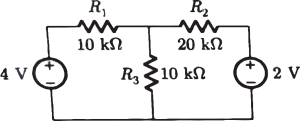(a) R_1
(b) R_2
(c) R_3
(d) both R_1 and R_3

Q7. The transfer function for a single loop non-unity feedback control system is
G(S)=1/(s^2+s+2),H(S)=1/(s+1)
The steady state due to unit step input is
(a) 6/7
(b) 6/5
(c) 2/3
(d) 0

Q8. The steady-state error of the system can be minimized by
(a) decreasing gain k
(b) increasing gain k
(c) reducing the oscillating frequency
(d) increasing the settling time

Q9. A 4 KVA, 400 V/200 V Single phase transformer has resistance of 0.02 p.u. and reactance of 0.06 pu. The resistance and reactance referred to HV side are
(a) 0.2 Ω and 0.6 Ω
(b) 0.8 Ω and 2.4 Ω
(c) 0.08 Ω and 0.24 Ω
(d) 2 Ω and 6 Ω

Q10. A three stage Johnson counter ring in figure is clocked at a constant frequency of f_c from starting state of Q_0 Q_1 Q_2=101. The frequency of output Q0 Q1 Q2 will be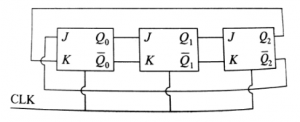(a) f_c/2
(b) f_c/3
(c) f_c/6
(d) f_c/8

SOLUTIONS

S1. Ans.(c)

Sol. Bending in provided over to the metallic sheet in and an underground cable. Hence statement (I) is true.

The purpose of bedding is to protect the sheath from mechanical injury due to due to armoring. You can think a shock absorber and hence statement (II) is false.

S2. Ans.(d)

Sol. Given data

Conductor diameter (2r = d) = 1cm

Insulation thickness (t) = 1.5 cm

Test voltage (V) = 33 kV

Hence D = 2R = 2r + 2t = d+ 2t = 4 cm

Maximum field strength is given as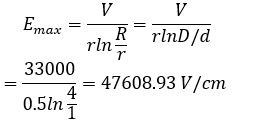S3. Ans.(c)

Sol.

 Bust type Specific quantities Unknown quantity Slack bus |V|, δ P, Q Load bus P, Q |V|, δ Controlled bus P, |V| Q, δ

S4. Ans.(a)

Sol. Given data;

Frequency = 50Hz, poles = 4

Speed (N) = 1425 rpm, rotor resistance  = 7.8Ω

Slip due to forward field  =

∵ Rotor resistance due to backward field =` r2/2(2-Sf )`
=7.8/2(2-0.05)
=2Ω

S5. Ans.(d)
Sol.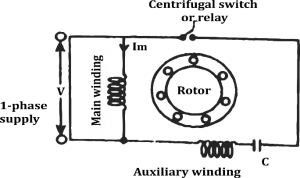In a split phase capacitor start induction motor phase difference between currents of the main and auxiliary winding is achieved by introducing capacitive reactance in the auxiliary winding circuit. Since

S6. Ans.(b)
Sol.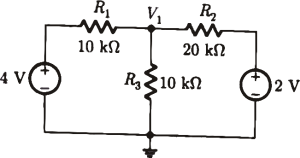Node equation at the center node
(V_1-4)/10+V_1/10+(V_1-2)/20=0
2V_1-8+2V_1+V_1-2=0
5V_1-10=0
V_1=2 volt
Voltage across R_2, V_R2=V_1-2=0
So, power absorbed by R_2 is zero.

S7. Ans.(c)
Sol. Steady state error due to step input is
`e_ss=A/(1+k_p )
k_p = lim┬(s→0)⁡G(S)H(S)
Where, k_P = static position error constant
Now given,
G(S)=1/(s^2+s+2) H(S)=1/(s+1) & A=1
k_P= lim┬(S→0)⁡〖1/((s^2+s+2) )〗 1/((S+1) )`
⇒ k_p=1/2
e_ss=1/(1+1/2)=2/3

S8. Ans.(b)
Sol. Steady state error (e_es ) α 1/k
So, as the gain of the system increases, steady-state error (e_es ) decrease.

S9. Ans.(b)
Sol. Z_b=(V_b^2)/(VA)_b =(400)^2/4000=40 Ω
∴R_2=0.02 ×40=0.8 Ω
And, X_2=0.06×40=2.4 Ω
NOTE: PU quantity will be same on both side.

S10. Ans.(c)
Sol. Johnson counter is mod 2N counter, where N=number of FF’s
∴output frequency (f_0 )=f_c/2N=f_C/(2×3)=f_c/6

×Join India's largest learning destination

What You Will get ?

•Job Alerts
•Daily Quizzes
•Subject-Wise Quizzes
•Current Affairs
•Previous year question papers
•Doubt Solving session

ORJoin India's largest learning destination

What You Will get ?

•Job Alerts
•Daily Quizzes
•Subject-Wise Quizzes
•Current Affairs
•Previous year question papers
•Doubt Solving session

ORJoin India's largest learning destination

What You Will get ?

•Job Alerts
•Daily Quizzes
•Subject-Wise Quizzes
•Current Affairs
•Previous year question papers
•Doubt Solving session

Enter the email address associated with your account, and we'll email you an OTP to verify it's you.Join India's largest learning destination

What You Will get ?

•Job Alerts
•Daily Quizzes
•Subject-Wise Quizzes
•Current Affairs
•Previous year question papers
•Doubt Solving session

Enter OTP

Please enter the OTP sent to
/6

Did not recive OTP?

Resend in 60sJoin India's largest learning destination

What You Will get ?

•Job Alerts
•Daily Quizzes
•Subject-Wise Quizzes
•Current Affairs
•Previous year question papers
•Doubt Solving sessionJoin India's largest learning destination

What You Will get ?

•Job Alerts
•Daily Quizzes
•Subject-Wise Quizzes
•Current Affairs
•Previous year question papers
•Doubt Solving session

Almost there

+91Join India's largest learning destination

What You Will get ?

•Job Alerts
•Daily Quizzes
•Subject-Wise Quizzes
•Current Affairs
•Previous year question papers
•Doubt Solving session

Enter OTP

Please enter the OTP sent to Edit Number

Did not recive OTP?

Resend 60

By skipping this step you will not recieve any free content avalaible on adda247, also you will miss onto notification and job alerts

Are you sure you want to skip this step?

By skipping this step you will not recieve any free content avalaible on adda247, also you will miss onto notification and job alerts

Are you sure you want to skip this step?